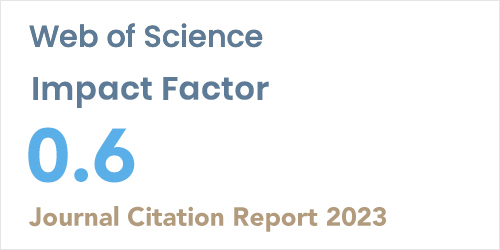ISSN: 1304-7191 | E-ISSN: 1304-7205
Volume: 41 Issue: 4 Year: 2023Application of crank-nicolson method to a random component heat equation
1Gümüşhane University, Dept. of Mathematical Engineering, GÜMÜŞHANE
2Karadeniz Teknik University, Department of Mathematics, TRABZON
Sigma J Eng Nat Sci 2020; 38(1): 475-480

#### Abstract

In this study, the solution of a random component heat equation is obtained by using Crank-Nicolson Method. The initial condition of this equation is examined by Normal distribution. The expected value and variance of solution of this equation are obtained. Crank-Nicolson method is applied to analyze the solution of this equation. Also, the solution and the graphs of the expected value and variance are obtained by using MATLAB software. The results of the heat equation are compared with random characteristics of this equation. Firstly, a random component heat equation is solved by this method.